## First-Order Linear Equations

A first‐order differential equation is said to be linear if it can be expressed in the formwhere P and Q are functions of x. The method for solving such equations is similar to the one used to solve nonexact equations. There, the nonexact equation was multiplied by an integrating factor, which then made it easy to solve (because the equation became exact).

To solve a first‐order linear equation, first rewrite it (if necessary) in the standard form above; then multiply both sides by the integrating factor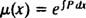The resulting equation,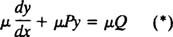is then easy to solve, not because it's exact, but because the left‐hand side collapses: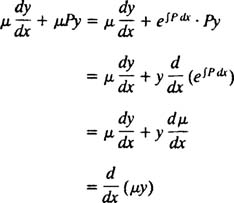Therefore, equation (*) becomes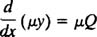making it susceptible to an integration, which gives the solution: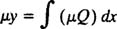Do not memorize this equation for the solution; memorize the steps needed to get there.

Example 1: Solve the differential equationThe equation is already expressed in standard form, with P(x) = 2 x and Q(x) = x. Multiplying both sides bytransforms the given differential equation into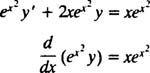Notice how the left‐hand side collapses into ( μy)′; as shown above, this will always happen. Integrating both sides gives the solution:Example 2: Solve the IVPNote that the differential equation is already in standard form. Since P(x) = 1/ x, the integrating factor is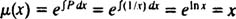Multiplying both sides of the standard‐form differential equation by μ = x givesNote how the left‐hand side automatically collapses into ( μy)′. Integrating both sides yields the general solution:Applying the initial condition y(π) = 1 determines the constant c: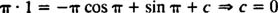Thus the desired particular solution isor, since x cannot equal zero (note the coefficient P(x) = 1/ x in the given differential equation),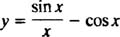Example 3: Solve the linear differential equation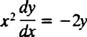First, rewrite the equation in standard form: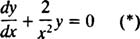Since the integrating factor here is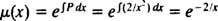multiply both sides of the standard‐form equation (*) by μ = e −2/ x ,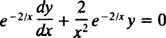collapse the left‐hand side,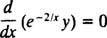and integrate: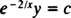Thus the general solution of the differential equation can be expressed explicitly asExample 4: Find the general solution of each of the following equations:

a.b.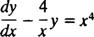Both equations are linear equations in standard form, with P(x) = –4/ x. Sincethe integrating factor will befor both equations. Multiplying through by μ = x −4 yields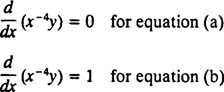Integrating each of these resulting equations gives the general solutions:Example 5: Sketch the integral curve of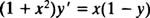which passes through the origin.

The first step is to rewrite the differential equation in standard form:Since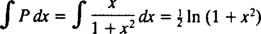the integrating factor isMultiplying both sides of the standard‐form equation (*) by μ = (1 + x 2) 1/2 gives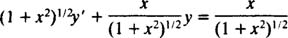As usual, the left‐hand side collapses into (μ y)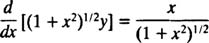and an integration gives the general solution: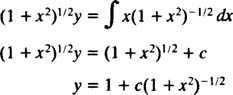To find the particular curve of this family that passes through the origin, substitute ( x,y) = (0,0) and evaluate the constant c:Therefore, the desired integral curve iswhich is sketched in Figure 1.Figure 1

Example 6: An object moves along the x axis in such a way that its position at time t > 0 is governed by the linear differential equation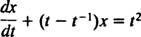If the object was at position x = 2 at time t = 1, where will it be at time t = 3?

Rather than having x as the independent variable and y as the dependent one, in this problem t is the independent variable and x is the dependent one. Thus, the solution will not be of the form “ y = some function of x” but will instead be “ x = some function of t.”

The equation is in the standard form for a first‐order linear equation, with P = tt −1 and Q = t 2. Since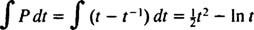the integrating factor is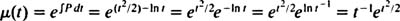Multiplying both sides of the differential equation by this integrating factor transforms it intoAs usual, the left‐hand side automatically collapses,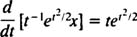and an integration yields the general solution:Now, since the condition “ x = 2 at t = 1” is given, this is actually an IVP, and the constant c can be evaluated:Thus, the position x of the object as a function of time t is given by the equationand therefore, the position at time t = 3 is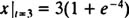which is approximately 3.055.